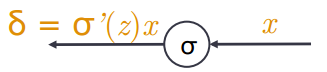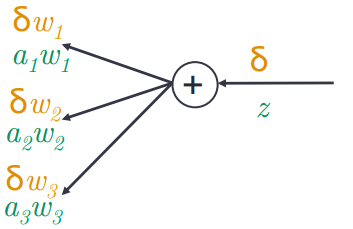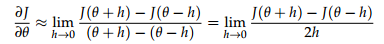# CS224d笔记3——神经网络

## 神经元

$$f(z)=\sigma(z)=\frac{1}{1+\exp(-z)}$$

$$\sigma'(z)=\sigma(z)(1-\sigma(z))$$

## Max-margin目标函数

$$\text{minimize}J=\max(s_c-s,0)$$

$$\text{minimize}J=\max(1+s_c-s,0)$$

## 后向传播（链式法则）

$$\frac{\partial J}{\partial s}=-\frac{\partial J}{\partial s_c}=-1$$

\begin{align*} \frac{\partial s}{\partial W_{ij}}&=\frac{\partial s}{\partial a_i}\frac{\partial a_i}{\partial z_i}\frac{\partial z_i}{\partial b_i}\\ &=U_if'(z_i)\frac{\partial (W_{i\cdot}x+b_i)}{b_i}\\ &=\delta_i \end{align*}

## 后向传播（误差传播）

1. 误差$$x$$向后传播穿过一个神经元时只需要将误差乘以当前神经元的局部梯度，如下图2. 误差$$\delta$$沿着线性变换后向传播时，只需要将误差乘以前向传播时的对应线性变换权重，如下图（绿色为前向，黄色为后向）1. 对于第k层特定的$$z_i^{(k)}$$处的误差首先需要传播到$$a_j^{(k-1)}$$位置，在前向计算时从$$a_j^{(k-1)}$$$$z_i^{(k)}$$是线性变换，所以$$a_j^{(k-1)}$$$$z_i^{(k)}$$处接收到的误差为$$\delta_i^{(k)}W_{ij}^{(k-1)}$$
2. 由于第k层每个$$z_i^{(k)}$$处的误差都会传播到$$a_j^{(k-1)}$$位置（层与层之间全连接）， 所以$$a_j^{(k-1)}$$从所有的$$z_i^{(k)}$$处收到的总误差为$$\sum_i \delta_i^{(k)}W_{ij}^{(k-1)}$$
3. 可以看到从$$z_j^{(k-1)}$$$$a_j^{(k-1)}$$穿过了一个神经元，所以$$a_j^{(k-1)}$$处的误差传播到$$z_j^{(k-1)}$$ 时需要乘以这个神经元的局部梯度$$f'(z_j^{(k-1)})$$，得到$$z_j^{(k-1)}$$处的误差$$\delta_j^{(k-1)}$$$$f'(z_j^{(k-1)})\sum_i \delta_i^{(k)}W_{ij}^{(k-1)}$$

$$\delta^{(k-1)}=f'(z^{(k-1)})\circ({W^{(k-1)}}^T\delta^{(k)})$$

## Tips和Tricks

### 神经网络的使用策略

1. 选择适当的网络结构
1. 结构：单个词，固定窗口，词袋，递归 vs 循环，CNN，基于句子 vs 基于文档
2. 非线性函数选择
2. 用梯度检查来校验是否有实现bug
3. 参数初始化
4. 优化技巧
5. 检查模型是否能够在数据集上过拟合
1. 如果不能，那么需要改变模型结果或者让模型参数规模更大（例如增加隐藏层）
2. 如果可以，那么增加正则化项

### 神经元非线性函数选择

$$\tanh(z)=2\sigma(2z)-1$$

sigmoid和tanh的函数图像和导数形式如下：tanh函数在深度网络中的表现通常要比sigmoid好，这里还有一些额外的非线性函数， 其中ReLu函数在2015年成为了深度神经网络最受欢迎的非线性函数。1. 实现前向计算和后向误差传播
2. 对于网络中的每一个参数（假设记作$$\theta$$$$\theta$$包含了网络所有的$$W$$$$b$$）， 然后按下列公式计算$$\theta$$每一维的梯度，$$h$$一般取一个较小的数（例如0.00001）：3. 对比后向误差传播计算的梯度和第2步计算的梯度是否很接近

### 参数初始化

$$W^{(l)}\sim U\left[-\frac{\sqrt{6}}{\sqrt{n^{(l)} + n^{(l+1)}}},\frac{\sqrt{6}}{\sqrt{n^{(l)}+n^{(l+1)}}}\right]$$

### 正则化（Regularization）

$$a^{(k+1)}=f\left (W^{(k)}(r\circ a^{(k)})+b\right )$$
$$p$$为超参，其中$$r$$的每一维以概率$$p$$置成1，以$$1-p$$的概率置成0。测试的时候不需要Dropout， 采用Dropout可以也防止模型对于特定的特征共现过拟合。